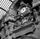cancel
Showing results for
Did you mean:Frequent Visitor

## How to set row criteria for Table.Min

Im using power query to lookup the min of TableA values for a custom column in TableB. I want to use criteria to only get the min value for each project, not the whole table. The value P1 should come from the current row of TableB.

How to do this? Seems like we need a MinX for Power Query.

= Table.Min(TableA, "MinStartDate", "ProjectName" = "P1")

2 ACCEPTED SOLUTIONSResolver IV

switch Table.Min by Table.MinN

see example...

let

Employees = Table.FromRecords(

{[Name="Bill", Level="P1", Salary=100000],
[Name="Barb", Level="P2", Salary=150000],
[Name="Andrew", Level="P3", Salary=85000],
[Name="Nikki", Level="P4", Salary=75000],
[Name="Margo", Level="P5", Salary=45000],
[Name="Jeff", Level="P6", Salary=200000]},

type table [
Name = text,
Level = number,
Salary = number
])

in

Table.MinN(Employees, "Salary", each [Level] ="P1")Frequent Visitor

Ah, Ive reread your reply from the first time round just now with a little more focus and it's exactly what I needed, thanks.
I missed the "each" in the criteria the first time through and so thought it was something I had already tried.

"each" provides the row context i was looking for.

UPDATE:

Actually no this doesn't work. I was looking at the wrong record. This just create a Record column on Table B, but every record is linked to "Project A". It ignores the Project of the current row. In the end Ive just gon with an left outer join and pared back the unneccesary columns.

5 REPLIES 5Anonymous
Not applicable

What you could try is creating a table that has the min and max for each project by using a group by, then use this resulting table for your criteria and pass each row of this into a function that will create the data you need.

If you wanted to get a single result of an entire table (different to what you want but an idea of patterns to use) and pass this to a function, then the code below demonstrates doing this and passing the result to a custom function.

let

minDate=Record.Field(Table.Min(#"factTable","factDate"),"factDate"),

maxDate=Record.Field(Table.Max(#"factTable","factDate"),"factDate"),

source=fnCreateCalendar(null,minDate,maxDate)

in

sourceResolver IV

switch Table.Min by Table.MinN

see example...

let

Employees = Table.FromRecords(

{[Name="Bill", Level="P1", Salary=100000],
[Name="Barb", Level="P2", Salary=150000],
[Name="Andrew", Level="P3", Salary=85000],
[Name="Nikki", Level="P4", Salary=75000],
[Name="Margo", Level="P5", Salary=45000],
[Name="Jeff", Level="P6", Salary=200000]},

type table [
Name = text,
Level = number,
Salary = number
])

in

Table.MinN(Employees, "Salary", each [Level] ="P1")Frequent Visitor

How are you setting the value "P1" though? Its a literal as written, whereas what I need is for it to look up the current Project Name of the current row in Table B.

So if P1 is a parameters then it can have a value of "Project A", Project B", etc depending on the row it is being evaluated against.Resolver IVFrequent Visitor

Ah, Ive reread your reply from the first time round just now with a little more focus and it's exactly what I needed, thanks.
I missed the "each" in the criteria the first time through and so thought it was something I had already tried.

"each" provides the row context i was looking for.

UPDATE:

Actually no this doesn't work. I was looking at the wrong record. This just create a Record column on Table B, but every record is linked to "Project A". It ignores the Project of the current row. In the end Ive just gon with an left outer join and pared back the unneccesary columns.